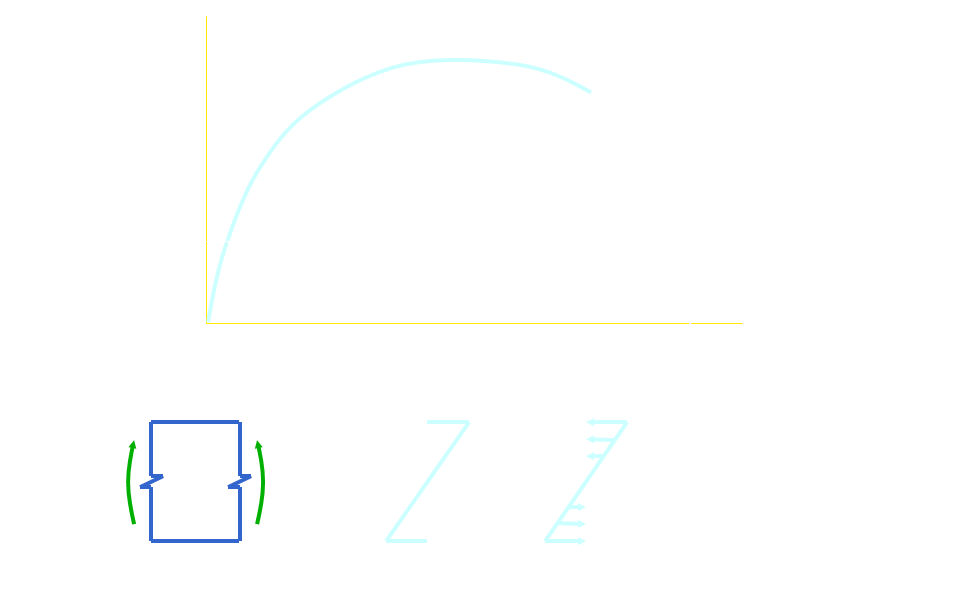# Introduction to Structural Concepts and Design

•Concrete behaviour varies depending on whether it is in tension or compression;
•The strength of concrete is given in terms of the characteristic strength;
•Characteristic strength is defined in AS 3600 as “the value of material strength, as assessed by standard test, which is exceeded by 95% of the material”;
•Characteristic strengths are denoted by the apostrophe;
•Standard characteristic compressive strengths are 20, 25, 32, 40 50 and 65 MPa.
•The characteristic flexural strength can be calculated using Equation 6.1.1.2, AS 3600:

f’cf = 0.6 (f’c)0.5

•Concrete strength is determined by testing a standard concrete cylinder in compression (cylinder is 200 mm high with a 100 mm diameter)
•Concrete stress-strain curve shows no definite yield point;
•Concrete does not have the large plastic deformation capacity of structural steel in the stress-strain curve, and so does not display the same ductile behaviour;
•Concrete has a brittle failure.
Pre-cracking Behaviour of Concrete
•When a concrete beam is sagging, the top of the beam is in compression and the bottom of the beam is in tension;
•If the tension in the bottom of the beam is less than the tensile capacity of the concrete, the concrete is able to carry the load;
•At relatively low strains (e.g. when cracking begins), the stress-strain relationship is approximately linear.•If the stress distribution is linear, the relationship between bending moment and stress is given by:

f = M y / I

where:  f =  bending stress;

M =  bending moment;

y =   distance from neutral axis to point;

I =  second moment of area of the section.

•The moment which causes the concrete in the tensile region to crack is called the cracking moment, Mcr.
•If the concrete has cracked, it can no longer take tensile load;
•The portion of the concrete in the tensile region which has cracked therefore has no contribution to the strength of the section.
•The solution adopted is to place reinforcing steel in the tensile region of the section to take the tensile loads.
•Individual bars are named with a letter and a number with the letter giving the type of bar while the number gives the diameter of the bar;
•In order to protect the reinforcing steel, the bars need a certain amount of ‘cover’;
•The amount of cover is specified in the concrete code, AS 3600, and depends on factors including concrete strength, location of the building, whether the member is interior or exterior etc;
•Cover is typically 20 – 50 mm.
Analysis of Concrete Sections
•So long as the stresses are low (elastic response), we ‘transform’ the entire section to concrete (these calculations were performed in Mechanics);
•Total compressive force = Total tensile force;
•Before cracking occurs the steel is taking tension, Ts, and so is the concrete, Tc;
•After cracking, only the steel is taking tension, Ts;
•All of the compression is taken by the concrete, Cc;

The moment carried by the section can be found by finding the moment of these forces about the neutral axis